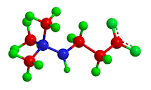## How to Calculate and Solve for the number of Particles in a Substance and Number of Moles | The Calculator EncyclopediaThe image above represent number of particles in a substance.

To compute for the number of particles in a substance, two essential paramters are needed and these parameters are Avogadro’s constant (h) and number of moles (n).

The formula for calculating the number of particles in a substance:

Number of particles in a substance = n x Avogadro’s constant

Where;
N  = Number of particles in a substance
n = Number of moles
h = Avogadro’s constant

Let’s solve an example:
Find the number of particles in a substance with an Avogadro’s constant of 6.022e+23 and number of moles of 12.

This implies that;
n = Number of moles = 12
h = Avogadro’s constant = 6.022e+23

Number of particles in the substance = 12 x 6.022e+23
Number of particles in the substance = 7.2264e+24

Therefore, the number of particles in the substance is 7.2264e+24 particles.